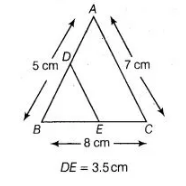# In ΔABC, AB = 5 cm,`
Question:

In ΔABC, AB = 5 cm, BC = 8 cm and CA = 7 cm. If D and E are respectively the mid-points of AB and BC, determine the length of DE.

Solution:

In ΔABC, we have AB = 5cm, BC = 8 cm and CA = 7 cm. Since, D and E are the mid-points of AB and BC, respectively.

By mid-point theorem, DE || AC

and

$D E=\frac{1}{2} A C=\frac{7}{2}=3.5 \mathrm{~cm}$